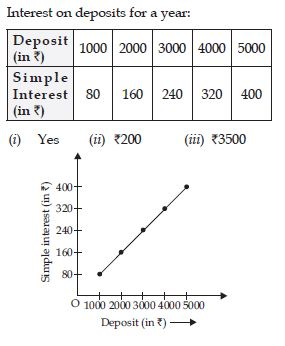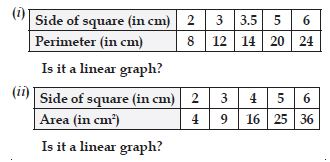# NCERT Solutions for Class 8 Math Chapter 15 - Introduction to Graphs

##### Question 1:

The following graph shows the temperature of a patient in a hospital, recorded every hour.
(a) What was the patient’s temperature at 1 p.m.?
(b) When was the patient’s temperature 38.5$°$ C?
(c) The patient’s temperature was the same two times during the period is given. What were these two times?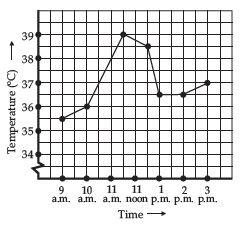(d) What was the temperature at 1.30 p.m.?
(e) During which periods did the patient’s temperature showed an upward trend?

(a) 36.5$°$C (b) 12 noon
(c) 1 p.m., 2 p.m.
(d) 36.5$°$C, the point between 1 p.m. and 2 p.m. on the x-axis is equidistant from the two points showing 1 p.m. and 2 p.m., so it will represent 1.30 p.m. Similarly, the point on the y-axis, between 36$°$C and 37$°$C will represent 36.5$°$C.
(e) 9 a.m. to 10 a.m., 10 a.m. to 11 a.m., 2 p.m. to 3 p.m.

##### Question 2:

The following line graph shows the yearly sales figures for a manufacturing company.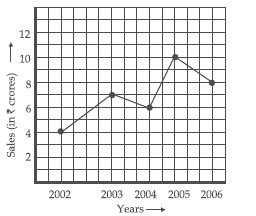(a) What were the sales in
(i) 2002 (ii) 2006
(b) What were the sales in:
(i) 2003 (ii) 2005
(c) Compute the difference between the sales in 2002 and 2006.
(d) In which year was there the greatest difference between the sales as compared to its previous year?

(a) (i) ₹4 crore
(ii) ₹8 crore
(b) (i) ₹7 crore
(ii) ₹10 crore
(c) ₹4 crore
(d) 2005

##### Question 3:

For an experiment in Botany, two different plants, Plant A and Plant B were grown under similar laboratory conditions. Their heights were measured at the end of each week for 3 weeks.
The results are shown by the following graph.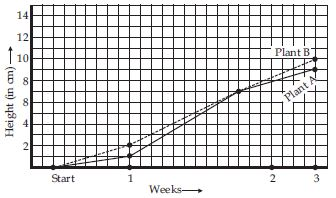(a) How high was Plant A after
(i) 2 weeks?
(ii) 3 weeks?
(b) How high was Plant B after
(i) 2 weeks?
(ii) 3 weeks?
(c) How much did Plant A grow during the 3rd week?
(d) How much did Plant B grow from the end
of the 2nd week to the end of the 3rd week?
(e) During which week did Plant A grow most?
(f) During which week did Plant B grow least?
(g) Were the two plants of the same height during any week shown here? Specify.

(a) (i) 7 cm (ii) 9 cm
(b) (i) 7 cm (ii) 10 cm
(c) 2 cm
(d) 3 cm
(e) Second week
(f) First week
(g) At the end of the 2nd week

##### Question 4:

The following graph shows the temperature
forecast and the actual temperature for each day of a week.
(a) On which days was the forecast temperature the same as the actual temperature?
(b) What was the maximum forecast temperature during the week?
(c) What was the minimum actual temperature during the week?
(d) On which day did the actual temperature differ the most from the forecast temperature?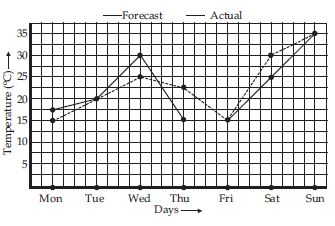(a) Tuesday, Friday, Sunday
(b) 35$°$C
(c) 15$°$C
(d) Thursday.

##### Question 5:

Use the tables below to draw linear graphs.
The number of days a hill side city received show in different years.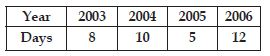The number of days a hill side city received show in different years.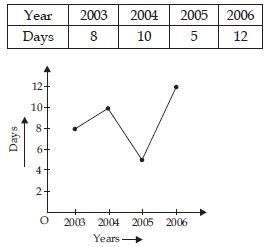Years shown along x-axis and days shown along y-axis.

##### Question 6:

Use the tables below to draw linear graphs.
Population (in thousands) of men and women in a village in different years: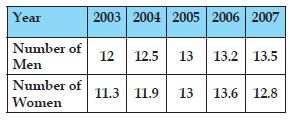Population (in thousands) of Men and Women in a village in different years: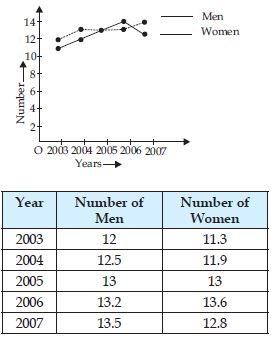##### Question 7:

A courier person cycles from a town to a neighbouring suburban area to deliver a parcel to a merchant. His distance from the town at different times is shown by the following graph: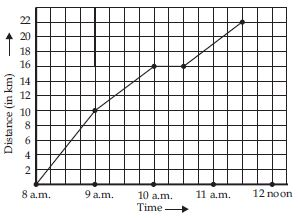(a) What is the scale taken for the time axis?
(b) How much time did the person take for the travel?
(c) How for is the place of the merchant from the town?
(d) Did the person stop on his way? Explain.
(e) During which period did he ride fastest?

(a) 4 units = 1 hour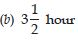(c) 22 km
(d) Yes, this is indicated by the horizontal part of the graph (10 a.m. – 10.30 a.m.)
(e) Between 8 a.m. and 9 a.m.

##### Question 8: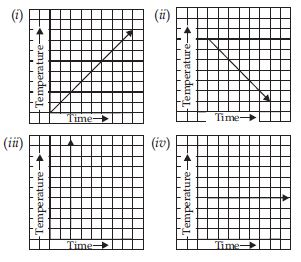(iii) is not possible.

##### Question 9:

Plot the following points on a graph sheet. Verify if they lie on a line.
(a) A (4, 0), B (4, 2), C (4, 6), D (4, 2.5)
(b) P (1, 1), Q (2, 2), R (3, 3), S (4, 4)
(c) K (2, 3), L (5, 3), M (5, 5), N (2, 5)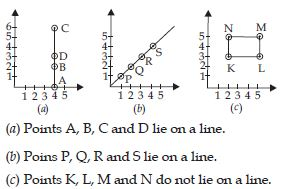##### Question 10:

Draw the line passing through (2, 3) and (3, 2). Find the coordinates of the points at which this line meets the x-axis and y-axis.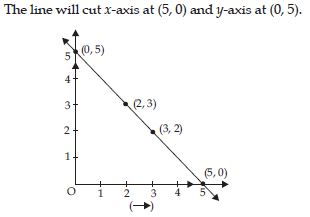##### Question 11:

Write the coordinates of the vertices of each of these adjoining figures.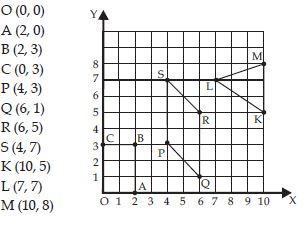##### Question 12:

State whether True or False. Correct that are false.
(i) A point whose x-coordinate is zero and y-co-ordinate is non-zero will lie on the y-axis.
(ii) A point whose y-coordinate is zero and x-coordinate is 5 will lie on y-axis.
(iii) The coordinates of the origin are (0, 0).

(i) True
(ii) False, (5, 0) will lie on x-axis.
(iii) True

##### Question 13:

In the graph, find how much petrol can be purchased for ₹800.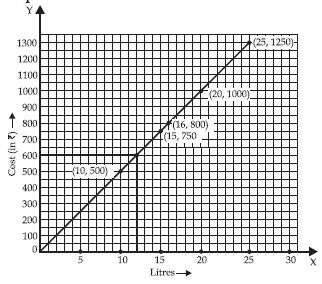##### Question 14:

Draw the graphs for the following tables of values, with suitable scales on the axes.
Cost of apples.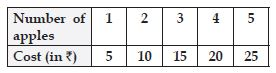The given table is: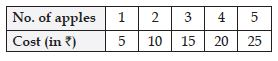The graph of the given table is as shown in the figure.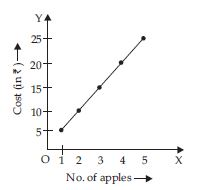##### Question 15:

Draw the graphs for the following tables of values, with suitable scales on the axes.
Distance travelled by a car.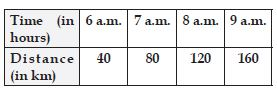(i) How much distance did the car cover during the period 7.30 a.m. to 8 a.m.?
(ii) What was the time when the car had covered a distance of 100 km, since it’s start?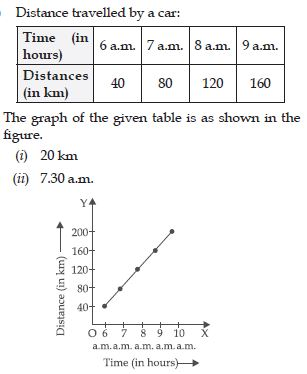##### Question 16:

Draw the graphs for the following tables of values, with suitable scales on the axes.
Interest on deposits for a year.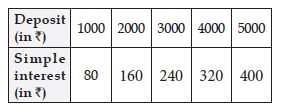(i) Does the graph pass through the origin?
(ii) Use the graph to find the interest on ₹2500 for a year.
(iii) To get an interest of ₹280 per year, how much money should be deposited.